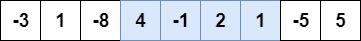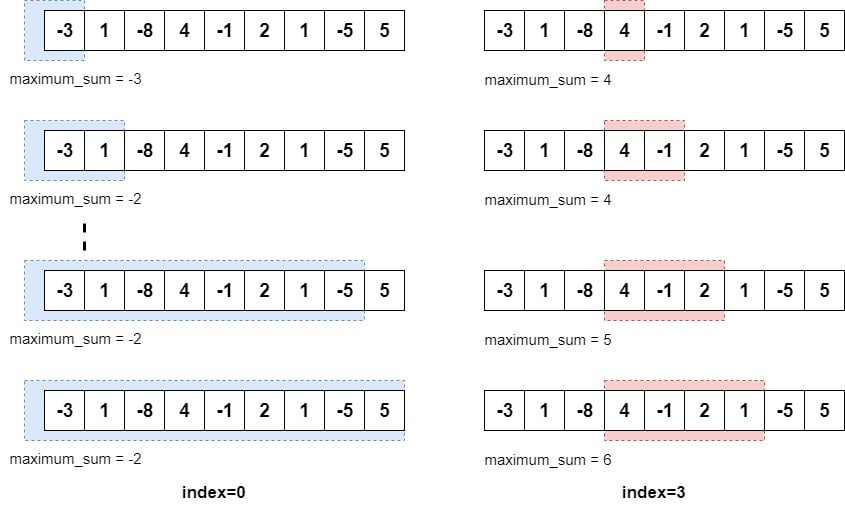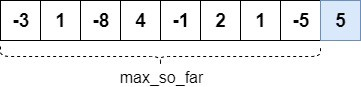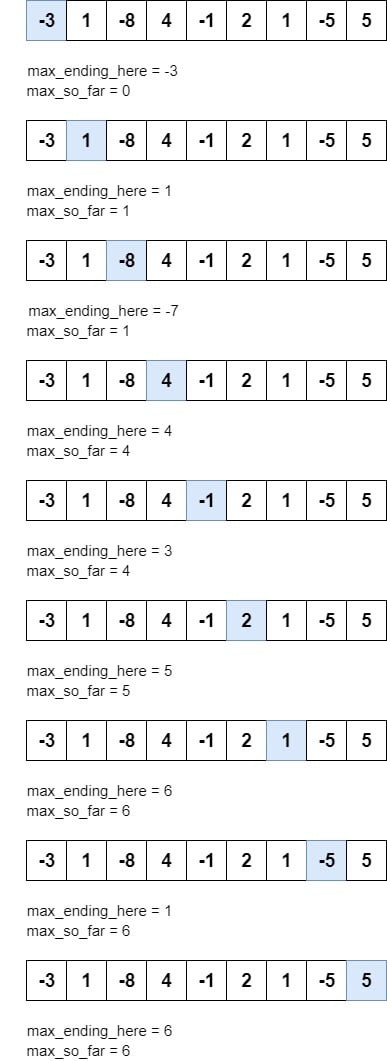### Get started with Spring and Spring Boot, through the Learn Spring course:

>> CHECK OUT THE COURSE

## 1. Overview

The maximum subarray problem is a task to find the series of contiguous elements with the maximum sum in any given array.

For instance, in the below array, the highlighted subarray has the maximum sum(6):In this tutorial, we’ll take a look at two solutions for finding the maximum subarray in an array. One of which we’ll design with O(n) time and space complexity.

## 2. Brute Force Algorithm

Brute force is an iterative approach to solve a problem. In most cases, the solution requires a number of iterations over a data structure. In the next few sections, we’ll apply this approach to solve the maximum subarray problem.

### 2.1. Approach

Generally speaking, the first solution that comes to mind is to calculate the sum of every possible subarray and return the one with the maximum sum.

To begin with, we’ll calculate the sum of every subarray that starts at index 0. And similarly, we’ll find all subarrays starting at every index from 0 to n-1 where n is the length of the array:So we’ll start at index 0 and add every element to the running sum in the iteration. We’ll also keep track of the maximum sum seen so far. This iteration is shown on the left side of the image above.

On the right side of the image, we can see the iteration that starts at index 3. In the last part of this image, we’ve got the subarray with the maximum sum between index 3 and 6.

However, our algorithm will continue to find all subarrays starting at indices between 0 and n-1.

### 2.2. Implementation

Let’s now see how we can implement this solution in Java:

``````public int maxSubArray(int[] nums) {

int n = nums.length;
int maximumSubArraySum = Integer.MIN_VALUE;
int start = 0;
int end = 0;

for (int left = 0; left < n; left++) {

int runningWindowSum = 0;

for (int right = left; right < n; right++) {
runningWindowSum += nums[right];

if (runningWindowSum > maximumSubArraySum) {
maximumSubArraySum = runningWindowSum;
start = left;
end = right;
}
}
}
logger.info("Found Maximum Subarray between {} and {}", start, end);
return maximumSubArraySum;
}``````

As expected, we update the maximumSubArraySum if the current sum is more than the previous maximum sum. Notably, we then also update the start and end to find out the index locations of this subarray.

### 2.3. Complexity

Generally speaking the brute force solution iterates over the array many times in order to get every possible solution. This means the time taken by this solution grows quadratically with the number of elements in the array. This may not be a problem for arrays of a small size. But as the size of the array grows, this solution isn’t efficient.

By inspecting the code, we can also see that there are two nested for loops. Therefore, we can conclude that the time complexity of this algorithm is O(n2).

In the later sections, we’ll solve this problem in O(n) complexity using dynamic programming.

## 3. Dynamic Programming

Dynamic programming solves a problem by dividing it into smaller subproblems. This is very similar to the divide-and-conquer algorithm solving technique. The major difference, however, is that dynamic programming solves a subproblem only once.

It then stores the result of this subproblem and later reuses this result to solve other related subproblems. This process is known as memoization.

Kadane’s algorithm is a popular solution to the maximum subarray problem and this solution is based on dynamic programming.

The most important challenge in solving a dynamic programming problem is to find the optimal subproblems.

### 3.2. Approach

Let’s understand this problem in a different way:In the image above, we assume that the maximum subarray ends at the last index location. Therefore, the maximum sum of subarray will be:

``maximumSubArraySum = max_so_far + arr[n-1]``

max_so_far is the maximum sum of a subarray that ends at index n-2. This is also shown in the image above.

Now, we can apply this assumption to any index in the array. For example, the maximum subarray sum that ends at n-2 can be calculated as:

``maximumSubArraySum[n-2] = max_so_far[n-3] + arr[n-2]``

Therefore, we can conclude that:

``maximumSubArraySum[i] = maximumSubArraySum[i-1] + arr[i]``

Now, since every element in the array is a special subarray of size one, we also need to check if an element is greater than the maximum sum itself:

``maximumSubArraySum[i] = Max(arr[i], maximumSubArraySum[i-1] + arr[i])``

By looking at these equations, we can see that we need to find the maximum subarray sum at every index of the array. Thus, we divided our problem into n subproblems. We can find the maximum sum at every index by iterating the array only once:The highlighted element shows the current element in the iteration. At every index, we’ll apply the equation derived earlier to calculate a value for max_ending_here. This helps us in identifying whether we should include the current element in the subarray or start a new subarray starting at this index.

Another variable, max_so_far is used to store the maximum subarray sum found during the iteration. Once we iterate over the last index, max_so_far will store the sum of the maximum subarray.

### 3.3. Implementation

Again, let’s see how we can now implement the Kadane algorithm in Java, following the above approach:

``````public int maxSubArraySum(int[] arr) {
int size = arr.length;
int start = 0;
int end = 0;
int maxSoFar = arr, maxEndingHere = arr;
for (int i = 1; i < size; i++) {
maxEndingHere = maxEndingHere + arr[i];
if (arr[i] > maxEndingHere) {
maxEndingHere = arr[i];
if (maxSoFar < maxEndingHere) {
start = i;
}
}
if (maxSoFar < maxEndingHere) {
maxSoFar = maxEndingHere;
end = i;
}
}
logger.info("Found Maximum Subarray between {} and {}", Math.min(start, end), end);
return maxSoFar;
}``````

Here, we updated the start and end to find the maximum subarray indices.

Note that we take Math.min(start, end) instead of start as the start index of the maximum subarray. This is because, if the array contains only negative numbers, the maximum subarray would be the largest element itself. In this case, if (arr[i] > maxEndingHere) will always be true. That is, the value of start is greater than the value of end.

### 3.4. Complexity

Since we only need to iterate the array once, the time complexity of this algorithm is O(n).

So as we can see, the time taken by this solution grows linearly with the number of elements in the array. Consequently, it is more efficient than the brute force approach we discussed in the last section.

## 4. Conclusion

In this quick tutorial, we’ve described two ways to solve the maximum subarray problem.

First, we explored a brute force approach and saw that this iterative solution resulted in quadratic time. Later, we then discussed the Kadane algorithm and used dynamic programming to solve the problem in linear time.

As always, the full source code of the article is available over on GitHub.

### Get started with Spring and Spring Boot, through the Learn Spring course:

>> CHECK OUT THE COURSE